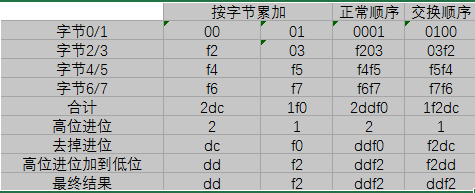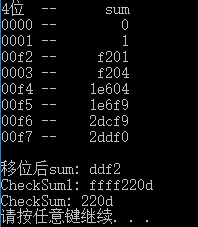﻿ 校验和计算原理_编程基础_编程_工业自动化网

# 校验和计算原理日期：2018-04-16     浏览：100    评论：0

# 校验和思路

``1、把校验和字段设置为0；  2、把需要校验的数据看成以16位为单位的数字组成，依次进行二进制反码求和；  3、把得到的结果存入校验和字段中 ``

``1、把首部看成以16位为单位的数字组成，依次进行二进制反码求和，包括校验和字段；  2、检查计算出的校验和的结果是否为0；  3、如果等于0，说明被整除，校验和正确。否则，校验和就是错误的，协议栈要抛弃这个数据包。 ``

# 校验和计算方法：

## 可结合性和可交换性

sum = [A，B]+’[C，D]+’[E，F]；

## 字节自主性

[A，B]+’[C，D]+’[E，F] = [B，A]+’（[D，C]+’[F，E]）

# 为什么使用二进制反码循环移位加法呢？

## 二进制反码循环移位加法求和优点

1. 不依赖系统是大端小端。即无论你是发送方计算机或者接收方检查校验和时，都不要调用htons或者ntohs，直接通过上面的算法就可以得到正确的结果。这个问题你可以自己举个例子，用反码求和时，交换16位数的字节顺序，得到的结果相同，只是字节顺序相应地也交换了；而如果使用原码或者补码求和，得到的结果可能就不同。

2. 计算和验证校验和比较简单、快递。

# 举例:## 代码：

``#include<iostream> #include<cstdio> #include<cstdlib> #include<cstring>  using namespace std;  void main(int argc, char *argv[]) {     FILE *fp;     char ch;     unsigned char LowChar, HighChar;     unsigned int count = 0, checksum = 0, byte = 0;     unsigned long int sum = 0;       //打开文件文本     if ((fp = fopen("I:\\1.txt", "r")) == NULL)     {         printf("File cannot be opened!");         system("pause");         return;     }     printf("4位  --      sum\n");     //从文本中读取字符     while(1)     {         if ((ch = fgetc(fp))!=EOF)         {             count++;                        //从1开始计数             if (ch != ' ')             {                 //将一个16进制字符转化为整形                 if (ch >= '0' && ch <= '9')                 {                     ch -= '0';                 }                 else if (ch >= 'a' && ch <= 'f')                 {                     ch = ch-'a'+10;                 }                 else if(ch >= 'A' && ch <= 'F')                 {                     ch = ch-'A'+ 10;                 }                   //计算8字节的累加值                 if (count%2 == 1)                 {                     HighChar = ch << 4;     //作为一个字节的高四位                 }                 else                 {                     LowChar = ch & 0x0f;    //作为一个字节的低四位                     byte = HighChar|LowChar;//构成一个字节                      //16位并行计算                     if (count % 4 == 2)     //高8位与sum相加                     {                         sum += byte << 8;                        }                     else if(count % 4 == 0) //低8位与sum相加                     {                         sum += byte;                     }                     printf("%04x -- %8lx    \n", byte, sum);                 }             }             else              {                 count--;             }         }         else         {             break;         }     }      //如果16位sum产生进位，将进位移加到低位     if ( sum >> 16)     {         checksum = ~(long(sum>>16)+long(sum&0x0000ffff));     }     printf("\n移位后sum: %x", (long(sum>>16)+long(sum&0x0000ffff)));     printf("\nCheckSum1: %x", checksum);     //避免再次进位      checksum = checksum&0x0000ffff;      //输出校验和     printf("\nCheckSum: %x\n", checksum);      system("pause"); }`1234567891011121314151617181920212223242526272829303132333435363738394041424344454647484950515253545556575859606162636465666768697071727374757677787980818283848586878889909192939495`

## 运行结果0相关评论

﻿